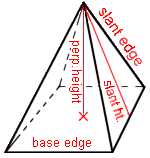Cleave Books
The Pyramids Calculator
 Show values to . . . 3 4 5 6 7 8 9 significant figures.
 base edge = units perp. height = unitsslant edge = units slant height = units volume = cubic units *f/b* angle = degrees
Remember: Appropriate units need to be attached.
For
*f/b* angle see notes below.
Very large and very small numbers appear in e-Format.
Unvalued zeros on all numbers have been suppressed.
The original inputs have NOT been adjusted in any way.
A note on Format and Accuracy is available.

 Additional InformationThe pyramid is probably known to most people through its associations with Egypt and the tombs of the pharaohs.It has a square base from which 4 triangular faces slope upwards to meet at a single point at the top known as the apex. The apex is vertically above the centre of the square base. Mathematically this is described as a right square-based pyramid (there are other sorts of pyramid) and is the one dealt with in this program.The perpendicular height is the height of the apex above the base.The slant height is the height measured along a triangular face, from the middle of a base-edge up to the apex. It is the perpendicular height of that triangle, and is needed if the area of one triangular face is to be found = one-half of base-edge x slant heightA slant edge is one of the 4 edges running from a corner of the base up to the apex, it is one of the edges of a triangular face. The *f/b* angle.This is the angle between one of the triangular faces and the base. It is also known as a dihedral angle.CautionsThe message that 'No solution was found' means only that. So, if a solution is possible then it was outside the limits set within this calculator.Also, in some cases, more than one solution might be possible, but only one (the first one found) is given here.

Go to Cleave Books main Index